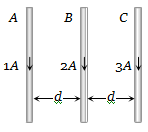There long straight wires A, B and C are carrying current as shown figure. Then the resultant force on B is directed(a) Towards A

(b) Towards C

(c) Perpendicular to the plane of paper and outward

(d) Perpendicular to the plane of paper and inward

(b) $F\propto {i}_{1}{i}_{2}$, so force on B due to C will be greater than that due A. Hence net force on B acts towards C.

Difficulty Level:

• 13%
• 63%
• 18%
• 8%